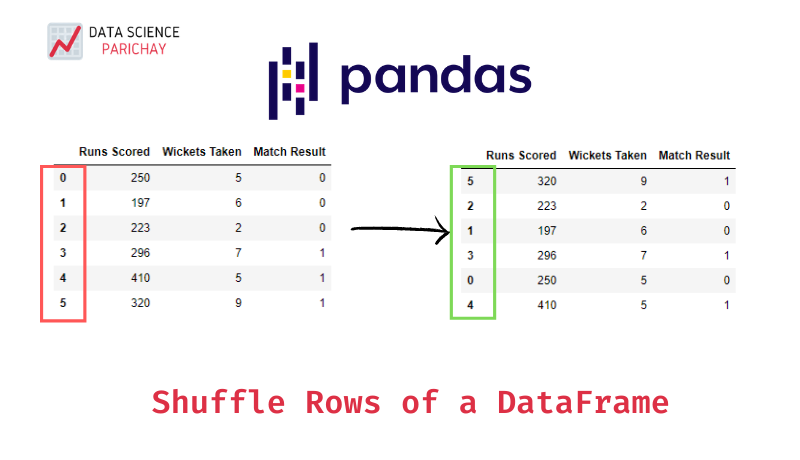# Randomly Shuffle Pandas DataFrame Rows

In this tutorial, we’ll look at how to randomly shuffle rows of a pandas dataframe. Suppose you have a dataframe with data that is ordered in some way. For example, the target variable for your classification task is in ascending order. And, you want to randomly shuffle the rows so that there’s a mix in the target variable values across the rows.

There are a number of ways to shuffle rows of a pandas dataframe. You can use the pandas `sample()` function which is used to generally used to randomly sample rows from a dataframe. To just shuffle the dataframe rows, pass `frac=1` to the function. The following is the syntax:

``df_shuffled = df.sample(frac=1)``

You can also use the `shuffle()` function from `sklearn.utils` to shuffle your dataframe. Here’s the syntax:

``````from sklearn.utils import shuffle
df_shuffled = shuffle(df)``````

Let’s look at some examples of using the above methods to shuffle rows of a dataframe. First, we’ll create the dataframe that we want to shuffle.

``````import pandas as pd

# Performance of a cricket team
df = pd.DataFrame({
'Runs Scored': [250, 197, 223, 296, 410, 320],
'Wickets Taken': [5, 6, 2, 7, 5, 9],
'Match Result': [0, 0, 0, 1, 1, 1]
})

# display the dataframe
print(df)``````

Output:

``````   Runs Scored  Wickets Taken  Match Result
0          250              5             0
1          197              6             0
2          223              2             0
3          296              7             1
4          410              5             1
5          320              9             1``````

The above dataframe shows the performance of a cricket team with its runs scored, wickets taken and the corresponding result in the match. Note that, the rows appear sorted on the result.

📚 Data Science Programs By Skill Level

Introductory

Intermediate ⭐⭐⭐

🔎 Find Data Science Programs 👨‍💻 111,889 already enrolled

Disclaimer: Data Science Parichay is reader supported. When you purchase a course through a link on this site, we may earn a small commission at no additional cost to you. Earned commissions help support this website and its team of writers.

A straightforward method to shuffle the rows. You can also give a seed value to the `random_state` parameter to make the results reproducible.

``````df_shuffled = df.sample(frac=1, random_state=0)
print(df_shuffled)``````

Output:

``````   Runs Scored  Wickets Taken  Match Result
5          320              9             1
2          223              2             0
1          197              6             0
3          296              7             1
0          250              5             0
4          410              5             1``````

The returned dataframe is shuffled. Also, note that the returned dataframe retains the index from the original dataframe. You can reset its index using the reset_index() function.

``````df_shuffled = df_shuffled.reset_index(drop=True)
print(df_shuffled)``````

Output:

``````   Runs Scored  Wickets Taken  Match Result
0          320              9             1
1          223              2             0
2          197              6             0
3          296              7             1
4          250              5             0
5          410              5             1``````

For more on the pandas `sample()` function, refer to its documentation.

For this, you’ll have to import the `shuffle()` function from the sklearn.utils module. The shuffle() function also has a `random_state` parameters to make your results reproducible.

``````from sklearn.utils import shuffle

# shuffle the dataframe
df_shuffled = shuffle(df, random_state=0)
print(df_shuffled)``````

Output:

``````   Runs Scored  Wickets Taken  Match Result
5          320              9             1
2          223              2             0
1          197              6             0
3          296              7             1
0          250              5             0
4          410              5             1``````

The returned dataframe is shuffled compared to the original dataframe. Here as well, the index of the original dataframe is retained which can be reset as we did in the previous example.

For more on the sklearn `shuffle()` function, refer to its documentation.

With this, we come to the end of this tutorial. The code examples and results presented in this tutorial have been implemented in a Jupyter Notebook with a python (version 3.8.3) kernel having pandas version 1.0.5 and sklearn version 0.23.1

•